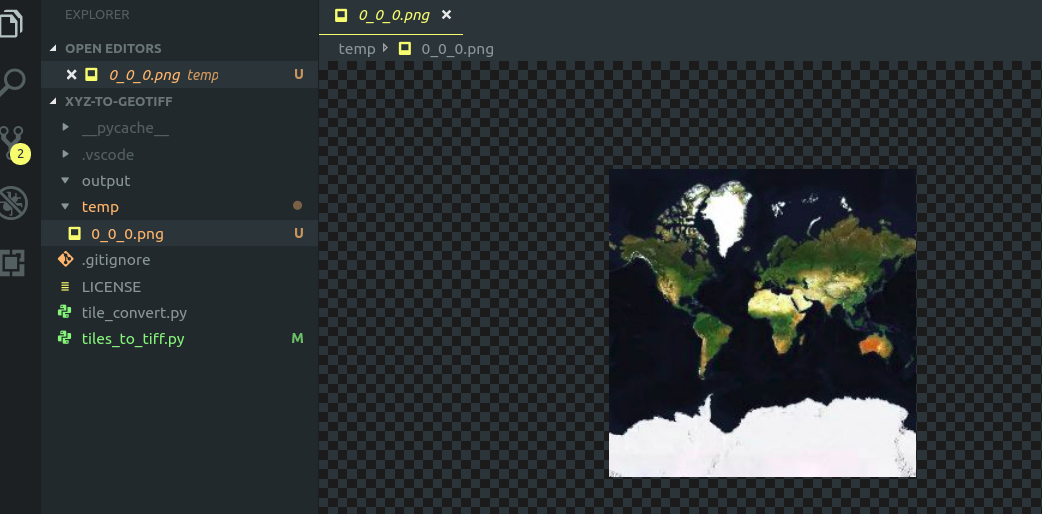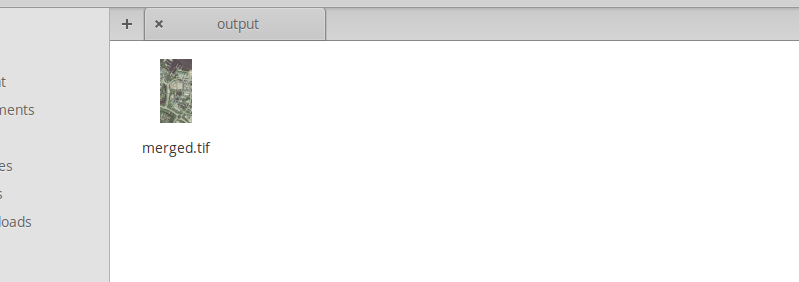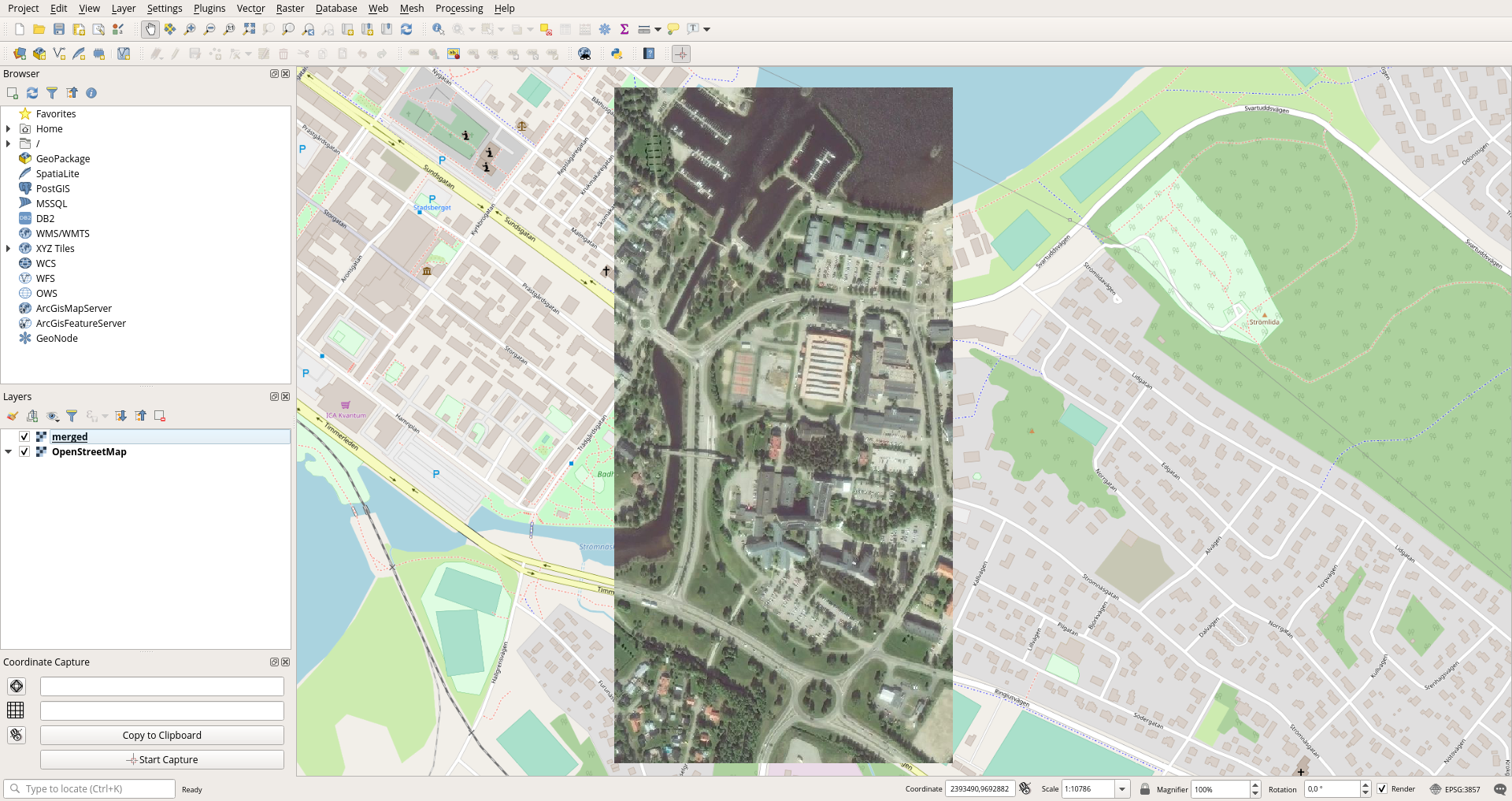# Generate merged GeoTIFF imagery from web maps (xyz tile servers) with Python

June 5, 2019

This guide will show you how to programmatically download map imagery from a web map (often referred to as slippy maps) tile server, georeference it and save it as a GeoTIFF. The GeoTIFF image can later be used for other purposes, in my case I used it to colorize a LIDAR data point cloud.

Prerequisites:

• Very basic Python knowledge (I'm really not a Python dev myself)
• GDAL with its Python bindings (if you're using Conda you can just follow along with the instructions below)

If you're just here looking for the finished source code you'll find it in this repo: https://github.com/jimutt/tiles-to-tiff

## Web maps and the XYZ tile format

Most online maps uses a shared way to render the map. Most maps are visualized by using multiple 256x256px raster tiles. The correct tiles are loaded by providing their x, y and z coordinates. Where z corresponds to the current zoom level.

For example Open Street Map tiles can be fetched with an URL following this pattern: https://a.tile.openstreetmap.org/{z}/{x}/{y}.png

The x, y and z parameters are integers and works as described on the OSM wiki:

X goes from 0 (left edge is 180 °W) to 2^zoom − 1 (right edge is 180 °E) Y goes from 0 (top edge is 85.0511 °N) to 2^zoom − 1 (bottom edge is 85.0511 °S) in a Mercator projection

For the curious, the number 85.0511 is the result of arctan(sinh(π)). By using this bound, the entire map becomes a (very large) square.

For a zoom level of n the number of tiles needed to cover the whole world is equal to 2^n × 2^n .

## Project setup

We'll be using Python together with GDAL which provide lots of convenient utilities for manipulation of geospatial raster data.

I'm using Conda to manage my Python environment as I find it to be very convenient for setting up isolated environments with easy installation of third party dependencies.

Create and activate a new Conda environment with gdal as a dependency:

``````conda create -n env_name gdal
conda activate env_name``````

## Convert latitude and longitude to corresponding tiles

Create a file called `tile_convert.py` for conversion from WGS84 coordinates to the corresponding tile at the specified zoom level (this fill will be containing various utility functions for transformation between WGS84 coordinates and tile names):

``````from math import log, tan, radians, cos, pi

def sec(x):
return(1/cos(x))

def latlon_to_xyz(lat, lon, z):
tile_count = pow(2, z)
x = (lon + 180) / 360
return(tile_count*x, tile_count*y)``````

In order to derive tile name from latitude and longitude a number of operations has to be executed. Combine the below steps and you'll end up with the `latlon_to_xyz` function:

1. Calculate the total number of tiles at the current zoom level: tile_count = 2^z
2. Reproject lat and lon to the Mercator projection (EPSG:3857). x = lon y = log(tan(lat) + sec(lat))
3. Transform x and y values to fit within the range of 0 - 1 and set origin to top left corner. x = (lon + 180) / 360 y = (1 − (y / π)) / 2
4. Multiply x and y by the number of tiles.

Next we'll add a new function (to the same file) which allows us to get the range of tiles for a specified rectangular area/bounding box:

``````def bbox_to_xyz(lon_min, lon_max, lat_min, lat_max, z):
x_min, y_max = latlon_to_xyz(lat_min, lon_min, z)
x_max, y_min = latlon_to_xyz(lat_max, lon_max, z)
return(floor(x_min), floor(x_max),
floor(y_min), floor(y_max))``````

Now we know how to calculate which individual tiles we need in order to cover a bounding box which corners are specified as WGS84 coordinates!

To download a map tile all you need to do is to send a GET request that matches the URL specification provided by the tile server. But make sure that you follow the user agreement/terms of service for the tile server you're accessing!

Create a new Python file called `tiles_to_tiff.py`. This will be our main script which will use download the required tiles, georeference each tile and then merge them into a single image.

``````import math
import urllib.request
import os

#---------- CONFIGURATION -----------#
tile_server = "https://api.mapbox.com/v4/mapbox.satellite/{z}/{x}/{y}.png?access_token=" + os.environ.get(
'MAPBOX_ACCESS_TOKEN')
temp_dir = os.path.join(os.path.dirname(__file__), 'temp')
output_dir = os.path.join(os.path.dirname(__file__), 'output')
zoom = 16
lon_min = 21.49147
lon_max = 21.5
lat_min = 65.31016
lat_max = 65.31688
#-----------------------------------#

url = tile_server.replace(
"{x}", str(x)).replace(
"{y}", str(y)).replace(
"{z}", str(z))
path = f'{temp_dir}/{x}_{y}_{z}.png'
urllib.request.urlretrieve(url, path)
return(path)``````

The `download_tile` function calls the specified tile server to download the requested tile and saves it as a PNG file in the output directory.

Create an empty "temp" folder in your workspace and add the below line to `tiles_to_tiff.py`:

``download_tile(0,0,0, tile_server)``

``\$ python tiles_to_tiff.py``

If you're able to execute the script successfully you should now see a 256x256px PNG image showing the whole world in the temp directory.Chances are high that you need a large image with higher resolution than what fits within a single tile. Therefore we'll use the `bbox_to_xyz` function to get the range of tiles needed and then we'll loop through every tile and pass it to the download function. This could be done with multiple parallel requests to make it more efficient, but for now it's being kept as simple as possible.

``````x_min, x_max, y_min, y_max = bbox_to_xyz(
lon_min, lon_max, lat_min, lat_max, zoom)

for x in range(x_min, x_max + 1):
for y in range(y_min, y_max + 1):
print(f"{x},{y}")

The above code will download all required tiles as standard, non georeferenced, PNG images. Later we are going to merge all tiles to a single picture and for that we'll use the handy gdal_merge.py script. Though it requires that we georeferenced the input imagery first.

## Georeferencing

In order to georeference the downloaded tiles we first need to reverse the translation we performed from latitude and longitude to the tile name format. We need to know the coordinates for each of the four corners of the tile. Then we'll use `gdal.Translate` to save the image as a TIFF file with embedded geolocation data.

If we remember the steps needed to go from WGS84 coordinates to the XYZ tile names we can also figure out how to retrieve the min and max longitude from a tile with the following function. Add the function to the `tile_convert.py` file.

``````def x_to_lat_edges(x, z):
tile_count = pow(2, z)
unit = 360 / tile_count
lon1 = -180 + x * unit
lon2 = lon1 + unit
return(lon1, lon2)``````

For the latitude conversion we'll use the same approach but an additional function is added to translate back from the Mercator projection:

``````def mercatorToLat(mercatorY):
return(degrees(atan(sinh(mercatorY))))

def y_to_lat_edges(y, z):
tile_count = pow(2, z)
unit = 1 / tile_count
relative_y1 = y * unit
relative_y2 = relative_y1 + unit
lat1 = mercatorToLat(pi * (1 - 2 * relative_y1))
lat2 = mercatorToLat(pi * (1 - 2 * relative_y2))
return(lat1, lat2)``````

With these functions in place we'll write a function that returns an array with the min and max longitude and latitude values in the same order as expected by the outputBounds parameter for the `gdal.Translate` method. Add the `tile_edges` function to `tile_Convert.py`.

``````def tile_edges(x, y, z):
lat1, lat2 = y_to_lat_edges(y, z)
lon1, lon2 = x_to_lon_edges(x, z)
return[lon1, lat1, lon2, lat2]``````

Finally we will write a `georeference_raster_tile` function in `tiles_to_tiff.py` and then call the function from the download loop:

``````def georeference_raster_tile(x, y, z, path):
bounds = tile_edges(x, y, z)
filename, extension = os.path.splitext(path)
gdal.Translate(filename + '.tif',
path,
outputSRS='EPSG:4326',
outputBounds=bounds)``````
``````for x in range(x_min, x_max + 1):
for y in range(y_min, y_max + 1):
print(f"{x},{y}")
georeference_raster_tile(x, y, zoom, png_path)``````

## Merge tiles to one large image

As we've already included a dependency to GDAL I chose to also use a GDAL python script to merge the tile images. We'll be invoking `gdal_merge.py` to do the work for us. You will need to add imports for `glob` and `subprocess` first.

``````def merge_tiles(input_pattern, output_path):
merge_command = ['gdal_merge.py', '-o', output_path]

for name in glob.glob(input_pattern):
merge_command.append(name)

subprocess.call(merge_command)``````

Create a folder called "output" in your workspace. Set the `lon_min`, `lon_max`, `lat_min` and `lat_max` to your liking as well as the `zoom`. I'm using these settings:

``````zoom = 15
lon_min = 21.49147
lon_max = 21.5
lat_min = 65.31016
lat_max = 65.31688``````

Add a call to the `merge_tiles` function at the bottom of `tiles_to_tiff.py`.

``````for x in range(x_min, x_max + 1):
for y in range(y_min, y_max + 1):
print(f"{x},{y}")
georeference_raster_tile(x, y, zoom, png_path)

print("Merging tiles")
merge_tiles(temp_dir + '/*.tif', output_dir + '/merged.tif')
print("Merge complete")``````

### Clear temp directory

Before we run the script and take a look at the results you might want to add an automatic cleanup of the temp folder. I'm doing it by importing `shutil` for removing the temp directory to then recreate it:

``````shutil.rmtree(temp_dir)
os.makedirs(temp_dir)``````

##Showtime! Now let's run the script and inspect the output. I'm using the bounding box specified earlier and aerial imagery from Mapbox.

``\$ python tiles_to_tiff.py ``

Output:

``````Downloading 8 tiles
36680,16917
36680,16918
36680,16919
36680,16920
36681,16917
36681,16918
36681,16919
36681,16920
When inspecting the "output" folder it now contains a single stitched TIFF image of the specified area.